The Mathematics of the 3D Rotation Matrix

# The Mathematics of the 3D Rotation Matrix

### Diana Gruber

Presented at the Xtreme Game Developers Conference, September 30-October 1, 2000, Santa Clara, California.

The set of special orthogonal matrices is a closed set. What does that mean, and why do we care?

A question like this is usually discussed only in an upper-division set theory class, which is a class for seniors majoring in math on the theoretical side. Not math for engineering or science, but math for its own sake. By the time you get to a set theory class, you have passed all the difficult classes. Geometry, trigonometry, calculus and differential equations are behind you. As Terry Pratchett might say, you have gone through mathematics and come out the other side.

In an upper division set theory class, you will consider a math fact such as "a set contains its elements". This fact will be given a fancy name, like "The Baire Category Theorem", and you will be asked to prove it. Since you are in the habit of following along (or you wouldn't have made it all the way through mathematics and out the other side), you know exactly what to do. You pull out a sharp pencil, and using the precise notation you were given earlier, you work out the proof in 4 or 5 lines. You are filled with a feeling of peace and confidence, as the rightness of the proof is crystal clear. Then you put the pencil away. You have finished your homework before your coffee has grown cold.

Meanwhile, your friends across the hall in the Comp Sci department are receiving their homework assignment: Write an operating system. From scratch. Due Tuesday. And those guys wondered why I majored in math.

In this class, I am not going to ask you to prove the Baire Category Theorem, or any similar observation of obvious properties from the field of set theory. We are going to take it on faith that the set of special orthogonal matrices is a closed set. We are not theoretical mathematicians, after all, we are software engineers. We are not concerned with the "why" so much as the "what is it good for". It turns out, the closed set of special orthogonal matrices is good for some very powerful things.

## A Review of 3D Graphics Matrices

I am going to assume that you have already encountered matrices as they apply to 3D graphics programming. If not, you may want to get that information from another source. There are plenty of people willing to write about the beginnings of 3D matrix math. What I am writing about here is the middle. To be specific, I want to talk about interesting properties of the rotation matrix. (Which happens, by coincidence, to be a special orthogonal matrix, the set of all of which is closed. Keep that in mind as we go along.)

So, to review, when changing the point of view in a 3D geometry system, you rotate and translate each point according to the current position and orientation of the person doing the viewing. This is sometimes called the camera position, or the point of view (POV). You can also rotate and translate objects within the 3D geometry, using a similar technique. Rotation and translation are usually accomplished using a pair of matrices, which we will call the Rotation Matrix (R) and the Translation Matrix (T). These matrices are combined to form a Transform Matrix (Tr) by means of a matrix multiplication. Here is how it is represented mathematically: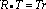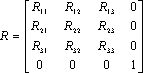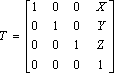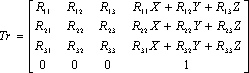There are other ways to represent this. The translation matrix is sometimes represented as a vector. It may be pre- or post- multiplied (changing between a right-handed system and a left-handed system). Sometimes the transform matrix has the translation elements at the bottom. Sometimes the last row is completely left off (especially in code because you don't really need it). I wrote the matrices this way because I find it convenient to multiply square matrices. If you are used to seeing it done some other way, you should be able to do the mental conversions without too much trouble.

## Properties of the Transform Matrix

Two features of Tr are obvious:

• It is very easy to extract the rotation matrix from the transform matrix
• It is a bit trickier to extract the translation matrix (or vector) from the transform matrix

Actually, it is not that hard to extract the translation matrix from the transform matrix. Just remember that IT = T where I is the identity matrix, and R-1R = I, so R-1RT = T, so R-1Tr = T. Since the inverse of an orthogonal matrix is its transpose (see below), RTTr = T. In other words, just multiply the transform matrix by the transpose of the rotation matrix to get the translation matrix. On second thought, it's tricky. Don't do it unless you have to. It will probably be easier to just keep a copy of the translation matrix.

The rotation matrix is easy get from the transform matrix, but be careful. Do not confuse the rotation matrix with the transform matrix. This is an easy mistake to make. When we talk about combining rotation matrices, be sure you do not include the last column of the transform matrix which includes the translation information. If you include that column, your matrix will no longer be a special orthogonal matrix, meaning it will no longer belong to the set which is closed, meaning you will not be able to count on it to produce the lovely results we are about to discover.

## Properties of the Rotation Matrix

Or, what is so special about a special orthogonal?

In case you missed it, a rotation matrix is a special orthogonal matrix. By definition, a special orthogonal matrix has these properties:

AAT = I

Where AT is the transpose of A and I is the identity matrix, and

det A = 1.

This isn't really very helpful. A more helpful set of properties is provided by Michael E. Pique in Graphics Gems (Glassner, Academic Press, 1990):

1. R is normalized: the squares of the elements in any row or column sum to 1.
2. R is orthogonal: the dot product of any pair of rows or any pair of columns is 0.
3. The rows of R represent the coordinates in the original space of unit vectors along the coordinate axes of the rotated space. (Figure 1).
4. The columns of R represent the coordinates in the rotated space of unit vectors along the axes of the original space.

Properties 1 and 2 are useful for verifying that a matrix is a rotation matrix. If you manipulate a matrix, and you want to make sure that you still have a rotation matrix, sum the squares of any row or column. If the result is not 1, then you have surely done something wrong. If the result is 1, chances are you are on the right track.

Property 3 is useful for forward motion. More about that later. Now that we have the formal properties of a rotation matrix, let's talk about the properties that apply, by convention, to 3D graphics programming.

## Rotation Matrix Conventions

Mathematically speaking, all special orthogonal matrices can be used as rotation matrices. But by convention, when we do 3D graphics programming, we designate special properties to the rows and columns.

In particular, we have names for the 3 rows of the rotation matrix. Row 1 is called Right, row 2 is called Up and row 3 is called Out, Forward, or View. I will call it "Out" because it represents the view looking outward from your eyes. It is, in fact, the unit vector describing the direction in which you are facing.

How does that work?Figure 1. The Out vector

See Figure 1. Suppose your point of view is at the origin, and you are looking out along a vector of undetermined length called View. The Out vector is a vector of length 1 which is parallel to View. The projection of Out onto the X, Y and Z axes is the third row of the rotation matrix. R31 is the projection of Out onto the X axis, R32 is the projection of Out onto the Y axis, and R33 is the projection of Out onto the Z axis.

You can verify property 1 above by taking the magnitude of the Out vector:

||Out|| = R312 + R322 + R332 = 1

The magnitude of Out is the sum of the squares of row 3 of the rotation matrix. (In general, you take the square root of the sum of the squares to get the magnitude of a vector. This is of course unnecessary in the case of a unit vector, because we know the magnitude is always going to be 1.)

That explains row 3 of the rotation matrix. What do the other rows represent? Very simply, they represent the other two axes of the rotated coordinate system. See Figure 2.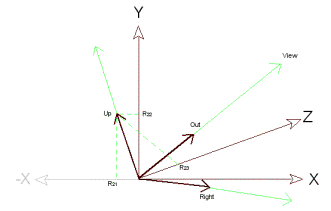Figure 2. The direction vectors of the rotated view

In Figure 2, the Up vector and the Right vector are displayed. Both are unit vectors, just like the Out vector. The projection of Up onto the X, Y and Z axes is the second row of the rotation matrix. In Figure 2, the Up projections are labeled R21, R22, and R23. The projection of Right is the first row of the rotation vector.

It seems we now have quite a lot of information. Is it enough information to construct a rotation matrix from scratch? Almost. There is one more problem to consider, and one more piece of information we need.

## Building a Rotation Matrix

Here is a common problem. Suppose you are a character in a game, and you are running around in the XZ plane. You hear a noise. You stop. A suspicion forms in your mind. Something is swooping down on you from above. Can it be? You look up slowly. The problem is illustrated in Figure 3.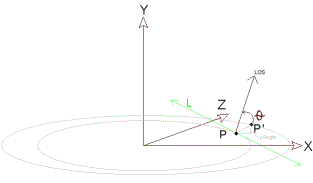Figure 3. Looking up slowly

Figure 3 shows the POV at point P in the XZ plane, facing point P'. You want to look up by angle(theta). Intuitively, you want to rotate around line L, which is tangent to the circle at point P which has its center at the origin. Perhaps you even know the rotation about the Y axis, which you may call yAngle. Isn't this enough information to build a rotation matrix R to describe the line of sight vector (LOS)?

No. Not quite. To see why, consider this. LOS is a vector which is normal (perpendicular) to a plane. The plane is what you are actually interested in looking at. It is a subset of the plane that will show up on your computer screen with a whole bunch of 3D objects projected onto it. While a normal to a plane tells us where the plane is and what directions it extends into, it does not tell us about the orientation of the plane.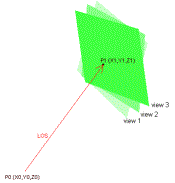Figure 4. Different views of the same plane

Figure 4 shows another picture of the same problem. In this case, you have a LOS vector defined by two points, P0 and P1. You are interested in a view of the plane that is normal to this vector at the P1 endpoint. As you can see, you have many views to choose from. The views in the plane represent the rotation around the LOS. You can only use one view. Clearly you need more information. How do you get it?

## The World Up Vector

The solution to the above problem turns out to be quite simple. You select another vector and use it as a frame of reference. This reference vector commonly lies on the Y axis and is sometimes called Up or Down. To avoid confusion with the Up vector I described earlier, which is a unit vector defining an axis of a rotated coordinate system, I will call this reference vector the World Up vector. This is also pretty standard. Furthermore, I will define the World Up vector to be (0,1,0). The shorthand for this vector is Upw.

Here is how it works.

The World Up vector is coplanar with the Up vector and the Out vector. Once you have your Out vector (the LOS described above) you still have an infinite number of Up vectors corresponding to the infinite number of rotated views. By requiring Up to be coplanar with Out and Upw, you are restricting Up to a single choice.

Remember, Up is also perpendicular to Out. Upw is probably not perpendicular to Up or Out, but it is coplanar with both. Let's have another look at the problem. See Figure 5.Figure 5. The new coordinate axes

In Figure 5 we have drawn unit vectors called Out, Up and Right, which correspond with the rotated coordinate axes. Out is parallel with the line of sight. Right is parallel to the tangent of the circle at point P. The circle lies in a plane that is perpendicular to Upw. Up is perpendicular to Out and Right, and it is coplanar with Out and Upw. Using this information, we can determine the coordinate axis projections of our Up vector.

There is a Direct3D function called D3Dutil_SetViewMatrix(). This function constructs a transform matrix given the information above. You pass two points (or vectors, as D3D prefers to call them), and you pass the World Up vector. I find it curious that Microsoft finds it necessary to pass the World Up vector. Apparently, they are expecting the vector to be changed. I can not think of a good reason to change the World Up vector. There are easier ways to rotate a view. Passing the World Up vector slows down the code, since it is necessary to perform validity checks each time the function is called. When designing Fastgraph, I assumed a fixed World Up vector. That doesn't mean you can't change it. It can be changed by calling the function fg_3Dupvector(). But I would expect that function to be called infrequently, if at all. In fact, using a unit vector superimposed on the Y axis as the World Up vector is such a good idea, I just can't think of any good reason to change it. Perhaps people who write flight simulators have a reason to change the World Up vector than I am not aware of. For now, and for the purposes of further discussion, we will assume a fixed World Up vector, as described above.

## Building a Rotation Matrix: Row 3

Now that we have all the ingredients, let's build and verify a rotation matrix. We will base this first rotation matrix on the LOS defined in Figure 4. We will start at the bottom and work up.

Row 3 of the rotation matrix is just the unit vector of the LOS projected onto the X, Y and Z axes. This is easy. You normalize the LOS by moving it to the origin and dividing by its magnitude or "norm". Do not confuse a norm with a normal. A norm is the magnitude of a vector. A normal is a vector that is perpendicular to a plane. If you have trouble with this, refer back to your primary reference on vector operations. Renaming the LOS to V, we get: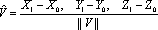whereThe caret signifies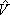is a unit vector, and is pronounced "V hat". I am not making this up.

So Row 3 of the rotation matrix is just this: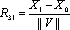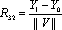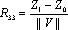Easy enough to code. Row 3 presents us with no problems. Tack a 0 on the end, and you have the third row of a rotation matrix.

## Building a Rotation Matrix: Row 2

Row 2 is the projection of Up onto the X, Y, and Z coordinate axes. We have a formula for this. The formula is: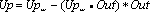That looks a little odd. I think we better verify that it works.

Take a look at Figure 6. Recall that by definition, a vector has magnitude and direction. The definition says nothing about position. That means we can put a vector anywhere we want, without changing its properties. In Figure 6, we choose to put Up, Upw and Out with their tails meeting at the origin. Also, we have decided these vectors must be coplanar, so we can look at them in 2D space. Up and Out are perpendicular. Out is separated from Upw by an angle, which may be any value, including 0.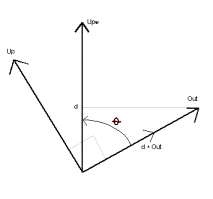Figure 6. Calculating the Up vector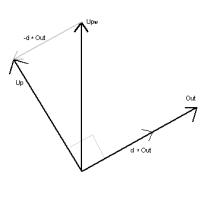Figure 7. The Up vector

It is clear from the diagram in Figure 6 that the projection of Out onto Upw is equal to the magnitude of Out times the cosine of . From the definition of vector dot product,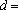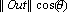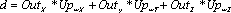The vector d*Out is just the vector in the direction of Out with magnitude d. You can subtract this from Upw as in Figure 7. By using similar triangles, it is easy to see the result is Up. Specifically: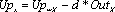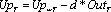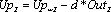Normalize Up before you put it in the rotation matrix. Do it the same way you normalized Out: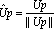You need to be careful here. If the magnitude of Up is 0 or close to 0, you will get a divide by 0 error when you try to normalize it. If that happens, use a different vector for Upw, such as a unit vector on another axis. (See the example source code.)

At last we have the second row of the rotation matrix: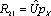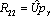## Building a Rotation Matrix: Row 1

We have worked our way up to the top of the rotation matrix. Compared to row 2, row 1 is easy. Since we want a unit vector that is perpendicular to both Up and Out, all we have to do is take the cross product of those two vectors. Since Up and Out are unit vectors, the result will be a unit vector. The only tricky thing now is deciding the order of the cross product. If you get it wrong, you will get a right-handed system where you wanted a left-handed system, or vice versa. For Fastgraph's left-handed coordinate system, I did the cross product this way: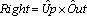So,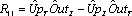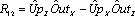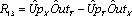And that takes care of our first rotation matrix.

## Other Ways to Build a Rotation Matrix

The method I just showed you is only one of several common ways to build a rotation matrix. There are other ways to do it. Perhaps the simplest rotation matrix is the one you get by rotating a view around one of the three coordinate axes. This is frequently documented and proved elsewhere, so I will just list the matrices here.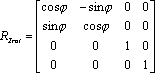Where,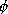(phi), and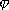(psi) are the rotations around the X, Y and Z axes. Notice these are the rotation matrices for a left handed system. To change them for a right handed system, just remember the sine function is an odd function, so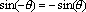Change the signs of all the sine terms to change the handedness.

There is one more way to build a matrix that I want to mention, but I won't derive it here because I want to get back to talking about the closed set of special orthogonal matrices. You can build a rotation matrix to rotate about any arbitrary axis like this: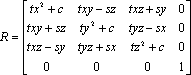Where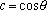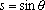and (x,y,z) is a unit vector on the axis of rotation. This matrix is presented in Graphics Gems (Glassner, Academic Press, 1990). I worked out a derivation in this article. Use this matrix to rotate objects about their center of gravity, or to rotate a foot around an ankle or an ankle around a kneecap, for example. It less useful for changing the point of view than the other rotation matrices. If you want, you can verify that rotating around a coordinate axis is a special case of this matrix. But I'll leave that to you. I'd rather get on with the good stuff.

## Properties of a Closed Set

Finally, I am ready to get to the point. The closed property of the set of special orthogonal matrices means whenever you multiply a rotation matrix by another rotation matrix, the result is a rotation matrix. That means you can combine rotations, and keep combining them, and as long as you occasionally correct for round-off error, you will always have a rotation matrix. In other words, you can use your current rotation matrix and the translation matrix to make relative changes to your position and view. Let's see how it works.

## Relative Rotation

Suppose you are at a some position P(X,Y,Z), and you are looking off in some direction D. Your position is represented by the translation matrix T, and the direction of your view is represented by the rotation matrix R. The combined information is held in the translation matrix Tr. We saw this at the beginning of the presentation: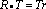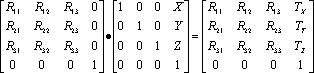Now suppose you want to look to the right. It is very easy. All you need to do is take the matrix for rotation around the Y axis and apply it to the transform matrix.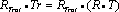Because of associative property of matrix multiplication,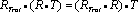And because the set of rotation matrices is closed,is guaranteed to be a rotation matrix.

But wait! We said we wanted to look to the right, no matter where we currently are or where we are currently looking. How does multiplying by RYrot make us look to the right? Isn't it just doing a rotation about the Y axis?

The answer is no. RYrot is performing a rotation around the Up vector. It just happens to be the Y axis when everything is at the default position (at the origin, staring down the Z axis). Once you have applied a transformation, all further rotations are relative to the new coordinate system. If you want to look up, apply a rotation around Right, or multiply by RXrot. If you want the screen in front of you to spin about a point in the center, rotate around Out, or multiply the transform by RZrot. These rotations correspond to Roll, Pitch, and Yaw which you have heard about. Roll is rotation about Out, Pitch is rotation about Right, and Yaw is rotation about Up. You can apply these to any transform matrix, and get a new transform matrix. And from that you will be able to extract a rotation matrix which is guaranteed to be a rotation matrix because the set of special orthogonal matrices is closed under multiplication. Okay, that's the last time I'll mention it.

## Relative Motion

Relative rotation, as discussed in the last section, is a powerful feature. The next feature I am going to mention is even more powerful. Actually, from a mathematical standpoint, it is probably equal. But I like it better. You probably will too. The next feature is relative motion.

Suppose you are writing a game, and you are in a 3D world, and you are facing some random direction, and you want to move straight ahead. How do you do it?

The answer lies in the third row of our good friend, the rotation matrix. If you remember from the previous discussion, the third row is the unit vector projection of the Out vector. It is the (x,y,z) components of a vector of length 1 pointing exactly in the direction you want to go. Let us suppose every time a key is pressed, you want your point of view to move forward by some amount n. All you have to do is take the elements of the third row, multiply each one by n, and add it to the appropriate elements in the translation matrix, as follows: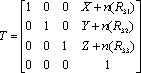That's it! Plug it in to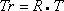and you have your new transform matrix. No need to calculate angles, or even know what they are. You can move forward based on row 3 of the rotation matrix because row 3 is guaranteed to have the properties of the third row of a rotation matrix. (You know why.)

Similarly, if you want to move to the right (strafe), use the values in row 1. If you want to move up, use the values in row 2. To move in the opposite directions, use negative values.

## An Optimization

So far, we've used the rotation matrix for relative rotation, and relative motion in either the forward direction or perpendicular to the line of sight. Is there anything else we can do with the rotation matrix? How about an optimization trick?

Suppose you want to render a large scene with zillions of polygons. Suppose you are moving through the scene, and you want to skip all the polygons that are behind the camera. Here is an easy way to detect and ignore points that have no possibility of being visible.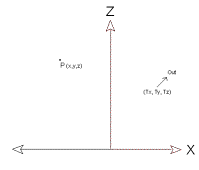Figure 8. A point P and the Out vector

Figure 8 shows the Out vector and a point P. How can we tell if P is behind the camera? It is very easy to do using the information in the rotation matrix and the translation matrix. To see how it works, draw a vector from the translated origin to the point P as in Figure 9.Figure 9. P is behind the camera

Figure 9 shows the Out vector and the P vector, along with the angle that q seperates them. It is easy to see from this diagram that P is definitely out of sight if cos() is negative. Remembering the definition of the vector dot product,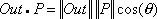you can see that cos() will be negative only when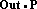is negative. This is a very simple calculation, compared to the work involved in rendering and projection. The dot product of the two vectors can be found like this: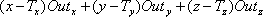Which in terms of our matrices looks like this: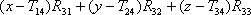If the value is positive, you had better render the point. If it is negative, you can safely skip it. Be careful to use the translation matrix, T, and not the transform matrix, Tr.

Sincegives you a magnitude as well as a vector, you can set a tolerance value other than 0. You can, for example, eliminate all points behind your z clipping limit. Or you can eliminate entire polygons, or groups of polygons (objects) based on a point in the center and a tolerance equal to the radius of the object. This can greatly reduce rendering time.

## An Example

No discussion of mathematics is complete without working a problem based on the theories under discussion. This problem will generate a rotation matrix from an LOS, then rotate the POV and generate a new rotation matrix, then verify that the matrix is a rotation matrix. Then we will generate a transform matrix and apply it to a point and verify that the results we get are the results we expect. This is a good example problem. You won't find a problem like this worked out in this much detail in very many places. And to show what good little mathematicians we are, we are going to work this problem without the benefit of a calculator. Are you ready? Let's go.

## The Problem

You are standing at a point (-1,0,1) and you are facing a point (-2,0,2). Generate a transform matrix for this view, then rotate the view upwards by 45 degrees. This is similar to the problem represented in Figure 3.

First, let's look at a 2D representation of the first part of the problem. Ignoring the Y axis (because the Y value is 0 for both points) let's draw a picture.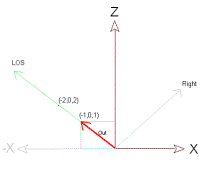Figure 10. The Out vector

Figure 10 shows the line of sight and the Out vector. Let's start by calculating and normalizing Out.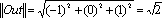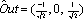That will go in row 3 of our rotation matrix. Next, we calculate Up and normalize it. This turns out to be trivial, and our Up corresponds with Upw.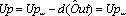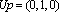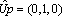Up will go into the second row of the rotation matrix. Finally, we calculate Right.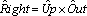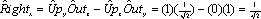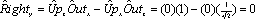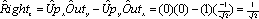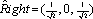That's all we need to make a rotation matrix! Here is what it looks like: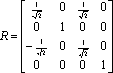Now we will make a transform matrix by plugging in the negative values of the point of view: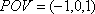Negative? Why negative? Because the transform is not going to be applied to the point of view. It is going to be applied to everything else. So if your point of view moves forward by 1, then everything else moves backwards by 1. I probably should have mentioned that before. Aren't you glad you bothered to work the problem? Okay, the transform matrix looks like this: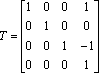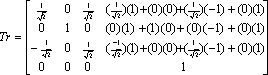That is the transform matrix for part one of the problem. We are now at (-1,0,1), looking at (-2,0,1). Part 2 has us looking up 45 degrees from here. To do that, we need to rotate around Right. We will use the RXrot matrix.Applying RXrot to R, we get a new rotation matrix, R':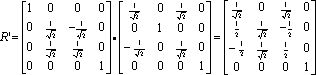Is R' a rotation matrix? We can verify it is orthogonal by multiplying by its inverse, which happens to be its transpose.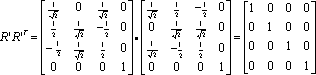That proves R' is an orthogonal matrix. To prove it is a special orthogonal matrix, you have to show the determinant is 1. I'll just skip that step for now.

Now we will build the final transform matrix. We do this by multiplying the translation matrix by the rotation matrix, as before.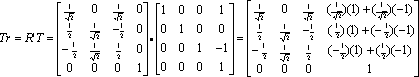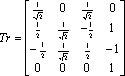And that is the final transform matrix. But how do we know it is right? Let's verify it by plugging in a point and see if we get the value we expect.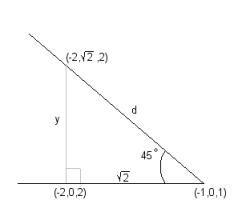Figure 11. Verifying a point

Look at the diagram in Figure 11. If you are looking up 45 degrees from the point (-1,0,1), you should be looking directly at the point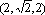. To verify this, calculate the distance between the two known points to get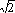. Using the law of sines, you can calculate the distance y.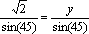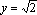You can also calculate the length of the hypoteneuse using the Pythagorean theorem: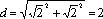Given this information, I would expect that if you applied the transform Tr to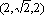you would end up with a point directly in front of you and 2 units away, or (0,0,2). Shall we try it?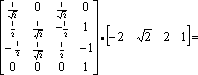= (0, 0, 2) which is what we wanted.

## Conclusion

That was a great example problem, wasn't it?

Oh, darn. We've run out of time. No time left to talk about quaternions. Are you as disappointed as I am? Don't worry, they're probably just a fad anyway. The real meat and potatoes of 3D graphics programming is all in the rotation matrix. Everything else is gravy. If you can understand the rotation matrix, you too can be a master 3D programmer.

See the handouts for more information and source code. Thank you for sitting through my presentation. Oh, and one more thing before I go. Remember how I said I was going to talk about how I did the 3D math in Fastgraph? I forgot to mention one thing. In Fastgraph, we wrote it in assembly language. See the handouts for a discussion of the advantages of performing concurrent operations with the floating point registers.

Good luck with your programming! And please visit my website some time: http://www.fastgraph.com.

Diana Gruber is Senior Programmer at Ted Gruber Software, Inc. and co-author of the Fastgraph programmer's graphics library.